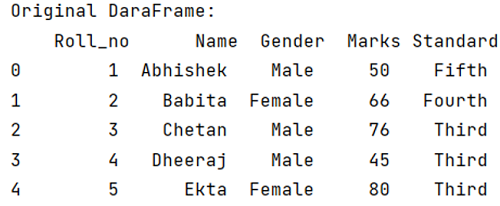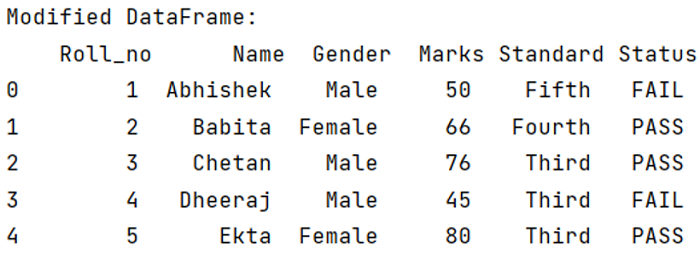# Python Pandas: Conditional creation of a series/DataFrame column

Given a DataFrame, we have to create a conditional column in a DataFrame.
Submitted by Pranit Sharma, on May 04, 2022

Columns are the different fields that contain their particular values when we create a DataFrame. We can perform certain operations on both rows & column values.

## Problem statement

Given a DataFrame, we have to create a conditional column in a DataFrame.

## Conditional creation of a series/DataFrame column

For this purpose, we use np.where() method. In this method, a condition is passed, and based on the condition it returns indices of elements in an input array which is also passed along with the condition.

### Syntax

```np.where('condition' [x,y])
```

### Parameter(s)

It takes a condition as a parameter, and two values, here X and Y, if the condition is true, it yields it value in X and otherwise Y.

Note

To work with Pandas and numpy, we need to install pandas and numpy packages first, below is the syntax:

```import numpy as np
import pandas as pd
```

Let us understand with the help of an example:

## Python program for conditional creation of a series/DataFrame column

```# Importing pandas package
import pandas as pd

# Creating a dictionary
d = {
'Roll_no': [ 1,2,3,4,5],
'Name': ['Abhishek', 'Babita','Chetan','Dheeraj','Ekta'],
'Gender': ['Male','Female','Male','Male','Female'],
'Marks': [50,66,76,45,80],
'Standard': ['Fifth','Fourth','Third','Third','Third']
}

# Creating a DataFrame
df = pd.DataFrame(d)

# Display original DataFrame
print("Original DataFrame:\n",df)
```

### Output

The output of the above program is:## Example 2

Now we will add another column named "Status" and set it as "PASS" if marks are greater than 60 and "FAIL" if marks are less than 60.

```# Importing pandas package
import pandas as pd
import numpy as np

# Creating a dictionary
d = {
'Roll_no': [ 1,2,3,4,5],
'Name': ['Abhishek', 'Babita','Chetan','Dheeraj','Ekta'],
'Gender': ['Male','Female','Male','Male','Female'],
'Marks': [50,66,76,45,80],
'Standard': ['Fifth','Fourth','Third','Third','Third']
}

# Creating a DataFrame
df = pd.DataFrame(d)

# Display original DataFrame
print("Original DataFrame:\n",df)

# Creating a new column on the basis
# of a condition
df['Status'] = np.where(df['Marks']>60, 'PASS','FAIL')

# Display modified DataFrame
print("Modified DataFrame:\n",df)
```

### Output

The output of the above program is: